# Rod Cutting Problem | (DP – 24)

Problem Statement:  Rod Cutting Problem

Problem Link: Rod Cutting Problem

We are given a rod of size ‘N’. It can be cut into pieces. Each length of a piece has a particular price given by the price array. Our task is to find the maximum revenue that can be generated by selling the rod after cutting( if required) into pieces.

```Example: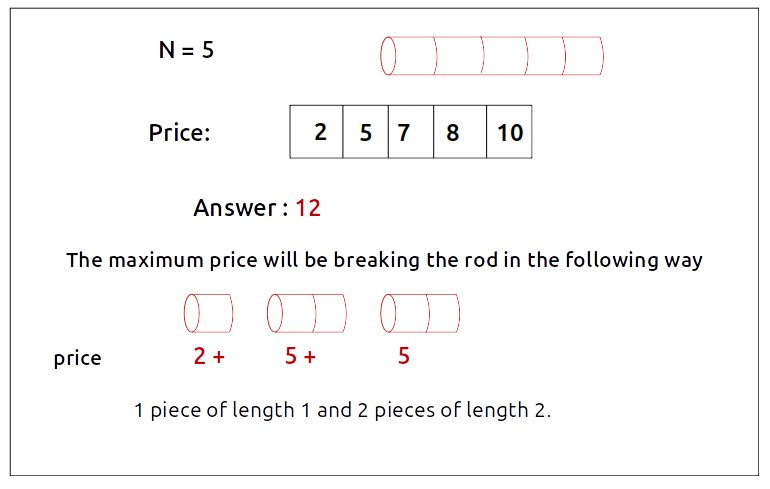```

Disclaimer: Don’t jump directly to the solution, try it out yourself first.

Pre-req: Unbounded Knapsack

### Intuition:

We want to cut the rod into pieces to get the maximum price. We can have 0 cuts as well if the whole rod is giving us a better price.
Suppose we have a rod of length 3, we will have 23-1 = 4 ways to cut the rod ( as we can cut the rod at (3-1) places and at every place we have 2 options, to cut it or not). The following figure shows all the ways: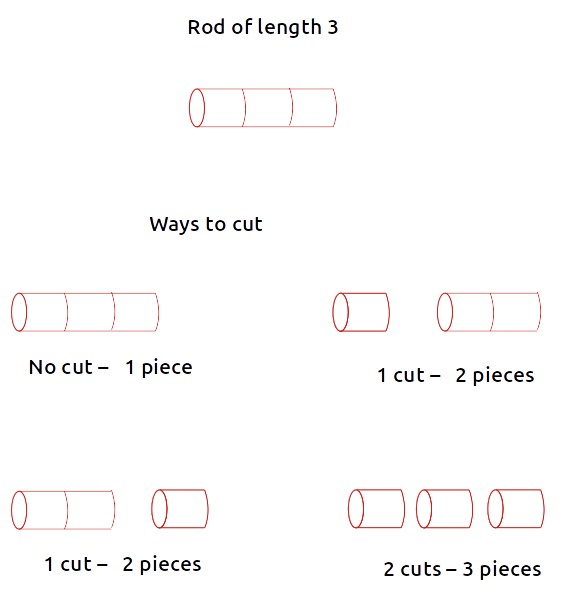The following two observations should be noted:

• We can’t have a rod piece bigger than the original rod length (self-explanatory). Therefore the rod length N acts like a limiting factor to the size of pieces we can cut.
• If we cut the piece of length ‘k’, we can still cut another piece from the remaining length of size ‘k’ again.

From the above two points, after a little thinking, we can say that this problem can be solved by the same technique we used in solving the Unbounded Knapsack

The rod pieces are equivalent to the items and the rod length is equivalent to the knapsack capacity. From where we will discuss the unbounded knapsack problem with the required modifications.

Steps to form the recursive solution:

We will first form the recursive solution by the three points mentioned in Dynamic Programming Introduction

Step 1: Express the problem in terms of indexes.

The price of individual lengths is given by the price array. Price gives us the price of a rod with length 1, index 2 gives us a rod with length 2, and so on. We can cut a rod from index 0 to  N-1. ( where the length of the rod will be ‘ind+1’). One parameter will be ‘ind’ which tells us the rod length that we want to cut from the original rod. Initially, we would want to consider the entire rod length.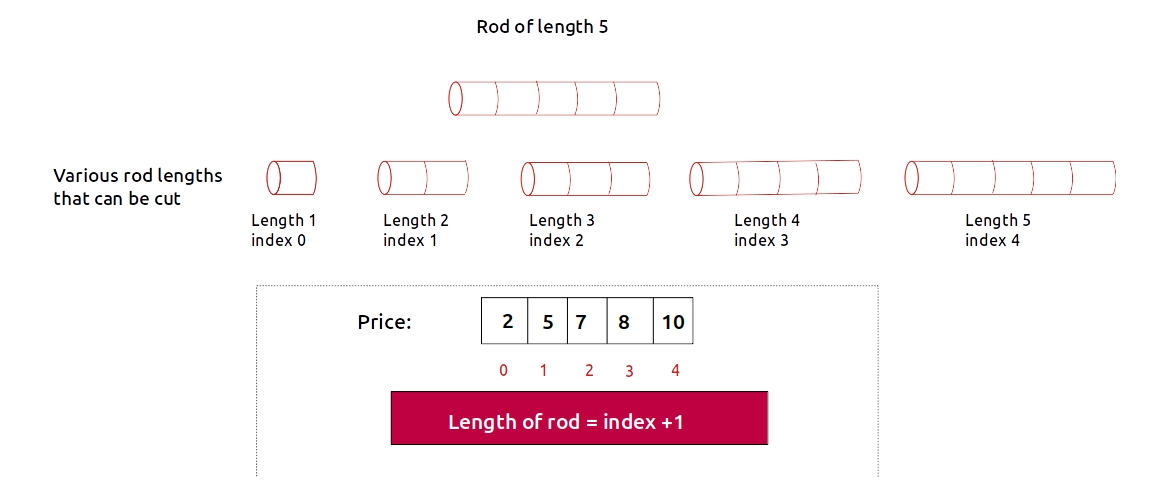We are given a rod with length ‘N’.So clearly another parameter will be ‘N’, i.e the total rod length that is given to us so that we can know the maximum length of rods that we can cut.

Initially, we would want to find f(N-1, N), i.e the maximum revenue generated by considering all rod lengths from index 0 to N-1 (i.e from length 1 to length N) with the total rod length given as N.

We can generalize this as: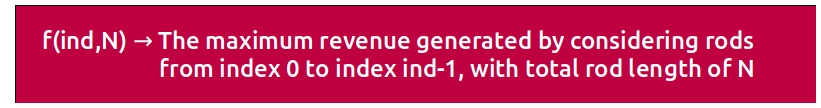Base Cases:

• If ind==0, it means we are considering a rod of length 1. Its price is given by price. Now for length N, the number of rod pieces of length 1 will be N (N/1). Therefore we will return the maximum revenue generated,i.e ‘N*price’.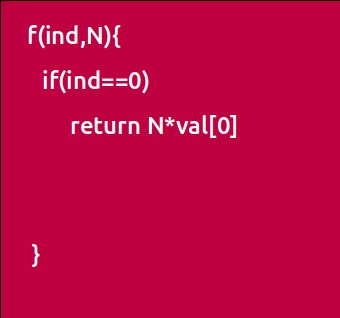Step 2: Try out all possible choices at a given index.

We need to generate all the subsequences. We will use the pick/non-pick technique as discussed in this video “Recursion on Subsequences”.

We have two choices:

• Exclude the current element in the subsequence: We first try to find a subsequence without considering the current index irod length. If we exclude the current item, the total length of the rod will not be affected and the revenue added will be 0 for the current item. So we will call the recursive function f(ind-1,N)
• Include the current element in the subsequence: We will try to find a subsequence by considering the current index rod length. As we have cut the rod, the total rod length will be updated to N-(ind+1), where ‘ind+1’ is the rod length, and the current rod piece price’s value (price[ind]) will also be added to the further recursive call answer.

Now here is the catch, we can cut the rod piece of the same index length. So we will not recursively call for f(ind-1, N-(ind+1)) rather we will stay at that index only and call for f(ind, N-(ind+1)) to find the answer.
Note: We will consider the current item in the subsequence only when the current element’s length is less than or equal to the total rod length ‘N’, if it isn’t we will not be considering it.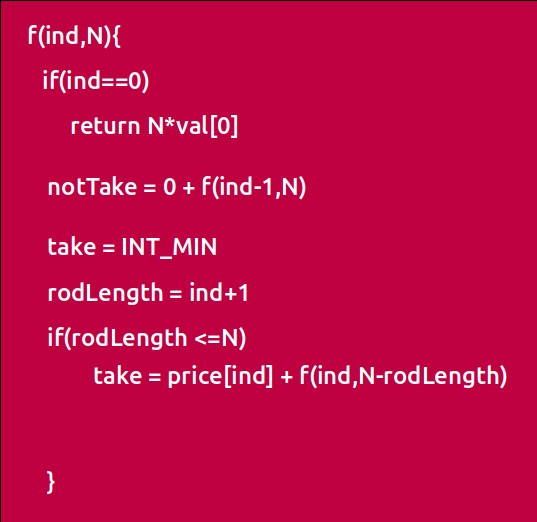Step 3:  Return the maximum of take and notTake

As we have to return the maximum amount of price we can generate, we will return the maximum of take and notTake.

The final pseudocode after steps 1, 2, and 3: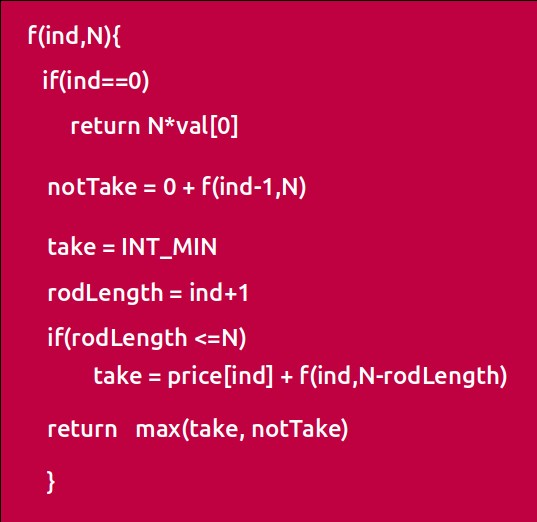Steps to memoize a recursive solution:

If we draw the recursion tree, we will see that there are overlapping subproblems. In order to convert a recursive solution the following steps will be taken:

1. Create a dp array of size [N][N+1]. The size of the price array is ‘N’, so the index will always lie between ‘0’ and ‘N-1’. The rod length can take any value between ‘0’ and ‘N’. Therefore we take the dp array as dp[N][N+1]
2. We initialize the dp array to -1.
3. Whenever we want to find the answer of particular parameters (say f(ind,N)), we first check whether the answer is already calculated using the dp array(i.e dp[ind][N]!= -1 ). If yes, simply return the value from the dp array.
4. If not, then we are finding the answer for the given value for the first time, we will use the recursive relation as usual but before returning from the function, we will set dp[ind][N] to the solution we get.

Code:

## C++ Code

``````#include <bits/stdc++.h>

using namespace std;

int cutRodUtil(vector<int>& price, int ind, int N, vector<vector<int>>& dp){

if(ind == 0){
return N*price;
}

if(dp[ind][N]!=-1)
return dp[ind][N];

int notTaken = 0 + cutRodUtil(price,ind-1,N,dp);

int taken = INT_MIN;
int rodLength = ind+1;
if(rodLength <= N)
taken = price[ind] + cutRodUtil(price,ind,N-rodLength,dp);

return dp[ind][N] = max(notTaken,taken);
}

int cutRod(vector<int>& price,int N) {

vector<vector<int>> dp(N,vector<int>(N+1,-1));
return cutRodUtil(price,N-1,N,dp);
}

int main() {

vector<int> price = {2,5,7,8,10};

int n = price.size();

cout<<"The Maximum price generated is "<<cutRod(price,n);
}
``````

Output:

The Maximum price generated is 12

Time Complexity: O(N*N)

Reason: There are N*(N+1) states therefore at max ‘N*(N+1)’ new problems will be solved.

Space Complexity: O(N*N) + O(N)

Reason: We are using a recursion stack space(O(N)) and a 2D array ( O(N*(N+1)).

## Java Code

``````import java.util.*;

class TUF{
static int cutRodUtil(int[] price, int ind, int N,int[][] dp){

if(ind == 0){
return N*price;
}

if(dp[ind][N]!=-1)
return dp[ind][N];

int notTaken = 0 + cutRodUtil(price,ind-1,N,dp);

int taken = Integer.MIN_VALUE;
int rodLength = ind+1;
if(rodLength <= N)
taken = price[ind] + cutRodUtil(price,ind,N-rodLength,dp);

return dp[ind][N] = Math.max(notTaken,taken);
}

static int cutRod(int[] price,int N) {

int dp[][]=new int[N][N+1];
for(int row[]:dp)
Arrays.fill(row,-1);
return cutRodUtil(price,N-1,N,dp);
}

public static void main(String args[]) {

int price[] = {2,5,7,8,10};

int n = price.length;

System.out.println("The Maximum price generated is "+cutRod(price,n));
}
}
``````

Output:

The Maximum price generated is 12

Time Complexity: O(N*N)

Reason: There are N*(N+1) states therefore at max ‘N*(N+1)’ new problems will be solved.

Space Complexity: O(N*N) + O(N)

Reason: We are using a recursion stack space(O(N)) and a 2D array ( O(N*(N+1)).

### Steps to convert Recursive Solution to Tabulation one.

To convert the memoization approach to a tabulation one, create a dp array with the same size as done in memoization. We can initialize it as 0.

• First we need to initialize the base conditions of the recursive solution.At ind==0, we are considering a unit length rod, so we will assign its value as (i * price), where i will iterate from 0 to N.
• Next, we are done for the first row, so our ‘ind’ variable will move from 1 to n-1, whereas our ‘length’ variable will move from 0 to ‘N’. We will set the nested loops to traverse the dp array.
• Inside the nested loops we will apply the recursive logic to find the answer of the cell.
• When the nested loop execution has ended, we will return dp[N-1][N] as our answer.

Code:

## C++ Code

``````#include <bits/stdc++.h>

using namespace std;

int cutRod(vector<int>& price,int N) {

vector<vector<int>> dp(N,vector<int>(N+1,-1));

for(int i=0; i<=N; i++){
dp[i] = i*price;
}

for(int ind=1; ind<N; ind++){
for(int length =0; length<=N; length++){

int notTaken = 0 + dp[ind-1][length];

int taken = INT_MIN;
int rodLength = ind+1;
if(rodLength <= length)
taken = price[ind] + dp[ind][length-rodLength];

dp[ind][length] = max(notTaken,taken);
}
}

return dp[N-1][N];
}

int main() {

vector<int> price = {2,5,7,8,10};

int n = price.size();

cout<<"The Maximum price generated is "<<cutRod(price,n);
}
``````

Output:

The Maximum price generated is 12

Time Complexity: O(N*N)

Reason: There are two nested loops

Space Complexity: O(N*N)

Reason: We are using an external array of size ‘N*(N+1)’. Stack Space is eliminated.

## Java Code

``````import java.util.*;

class TUF{

static int cutRod(int[] price,int N) {

int dp[][]=new int[N][N+1];

for(int row[]:dp)
Arrays.fill(row,-1);

for(int i=0; i<=N; i++){
dp[i] = i*price;
}

for(int ind=1; ind<N; ind++){
for(int length =0; length<=N; length++){

int notTaken = 0 + dp[ind-1][length];

int taken = Integer.MIN_VALUE;
int rodLength = ind+1;
if(rodLength <= length)
taken = price[ind] + dp[ind][length-rodLength];

dp[ind][length] = Math.max(notTaken,taken);
}
}

return dp[N-1][N];
}

public static void main(String args[]) {

int price[] = {2,5,7,8,10};

int n = price.length;

System.out.println("The Maximum price generated is "+cutRod(price,n));
}
}
``````

Output:

The Maximum price generated is 12

Time Complexity: O(N*N)

Reason: There are two nested loops

Space Complexity: O(N*N)

Reason: We are using an external array of size ‘N*(N+1)’. Stack Space is eliminated.

### Part 3: Space Optimization

If we closely look the relation,

dp[ind][length] =  max(dp[ind-1][length] ,dp[ind][length-(ind+1)])

We see that to calculate a value of a cell of the dp array, we need only the previous row values (say prev). So, we don’t need to store an entire array. Hence we can space optimize it.

We will be space optimizing this solution using only one row.

### Intuition:

If we clearly see the values required:  dp[ind-1][cap] and dp[ind-1][cap – wt[ind]], we can say that if we are at a column cap, we will only require the values shown in the blue box(of the same column) from the previous row and other values will be from the cur row itself. So why do we need to store an entire array for it?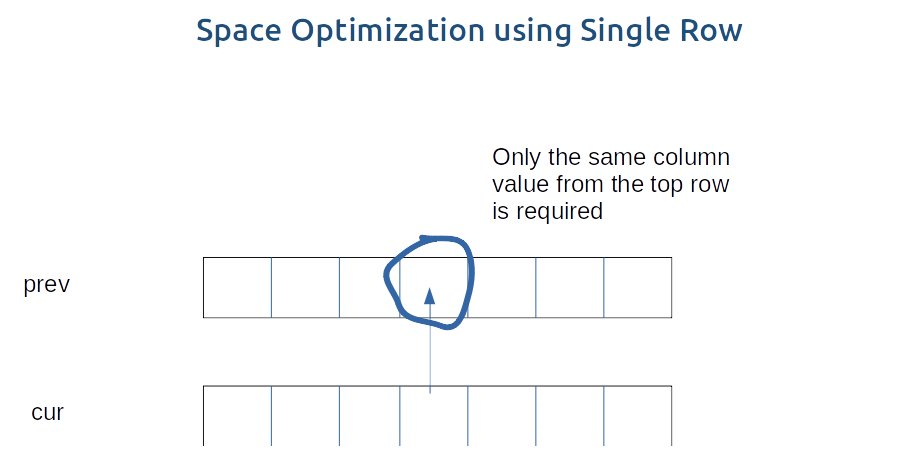If we need only one value from the prev row, there is no need to store an entire row. We can work a bit smarter.

We can use the cur row itself to store the required value in the following way:

• We somehow make sure that the previous value( say preValue) is available to us in some manner ( we will discuss later how we got the value).
• Now, let us say that we want to find the value of cell cur, by going through the relation we find that we need a preValue and one value from the cur row.
• We see that to calculate the cur element, we need only a single variable (preValue). The catch is that we can initially place this preValue at the position cur (before finding its updated value) and later while calculating for the current row’s cell cur, the value present there automatically serves as the preValue and we can use it to find the required cur value. ( If there is any confusion please see the code).
• After calculating the cur value we store it at the cur position so this cur will automatically serve as preValue for the next row. In this way we space optimize the tabulation approach by just using one row.

Code:

## C++ Code

``````#include <bits/stdc++.h>

using namespace std;

int cutRodUtil(vector<int>& price, int ind, int N, vector<vector<int>>& dp){

if(ind == 0){
return N*price;
}

if(dp[ind][N]!=-1)
return dp[ind][N];

int notTaken = 0 + cutRodUtil(price,ind-1,N,dp);

int taken = INT_MIN;
int rodLength = ind+1;
if(rodLength <= N)
taken = price[ind] + cutRodUtil(price,ind,N-rodLength,dp);

return dp[ind][N] = max(notTaken,taken);
}

int cutRod(vector<int>& price,int N) {

vector<int> cur (N+1,0);

for(int i=0; i<=N; i++){
cur[i] = i*price;
}

for(int ind=1; ind<N; ind++){
for(int length =0; length<=N; length++){

int notTaken = 0 + cur[length];

int taken = INT_MIN;
int rodLength = ind+1;
if(rodLength <= length)
taken = price[ind] + cur[length-rodLength];

cur[length] = max(notTaken,taken);
}
}

return cur[N];
}

int main() {

vector<int> price = {2,5,7,8,10};

int n = price.size();

cout<<"The Maximum price generated is "<<cutRod(price,n);
}
``````

Output:

The Maximum price generated is 12

Time Complexity: O(N*N)

Reason: There are two nested loops.

Space Complexity: O(N)

Reason: We are using an external array of size ‘N+1’ to store only one row.

## Java Code

``````import java.util.*;

class TUF{

static int cutRodUtil(int price[], int ind, int N, int[][] dp){

if(ind == 0){
return N*price;
}

if(dp[ind][N]!=-1)
return dp[ind][N];

int notTaken = 0 + cutRodUtil(price,ind-1,N,dp);

int taken = Integer.MIN_VALUE;
int rodLength = ind+1;
if(rodLength <= N)
taken = price[ind] + cutRodUtil(price,ind,N-rodLength,dp);

return dp[ind][N] = Math.max(notTaken,taken);
}

static int cutRod(int[] price,int N) {

int cur[]=new int[N+1];

for(int i=0; i<=N; i++){
cur[i] = i*price;
}

for(int ind=1; ind<N; ind++){
for(int length =0; length<=N; length++){

int notTaken = 0 + cur[length];

int taken = Integer.MIN_VALUE;
int rodLength = ind+1;
if(rodLength <= length)
taken = price[ind] + cur[length-rodLength];

cur[length] = Math.max(notTaken,taken);
}
}

return cur[N];
}

public static void main(String args[]) {

int price[] = {2,5,7,8,10};

int n = price.length;

System.out.println("The Maximum price generated is "+cutRod(price,n));
}
}
``````

Output:

The Maximum price generated is 12

Time Complexity: O(N*N)

Reason: There are two nested loops.

Space Complexity: O(N)

Reason: We are using an external array of size ‘N+1’ to store only one row.

Special thanks to Anshuman Sharma for contributing to this article on takeUforward. If you also wish to share your knowledge with the takeUforward fam, please check out this article